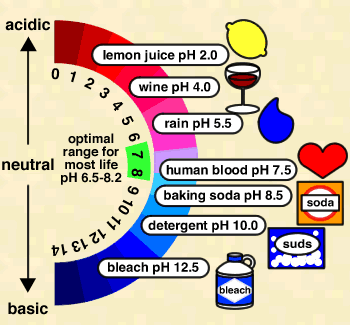## INFORMATION ON DRINKING WATER QUALITY OF THE INSTITUTEGeneral information
pH | Hardness | Alkalinity | Total Dissolved Solids | Fluoride | Nitrate | Sulfate | Arsenic | Chromium | Cadmium | Zinc | Iron | Lead | Copper | Chlorides | Turbidity | Bacteriological parametersHomeDrinking Water Quality StandardsWater quality resultsCommitteeAnalytical Methods

pHpH represents the effective concentration (activity) of hydrogen ions (H+) in water. This concentration could be expressed in the same kind of units as other dissolved species, but H+ concentrations are much smaller than other species in most waters. The activity of hydrogen ions can be expressed most conveniently in logarithmic units. pH is defined as the negative logarithm of the activity of H+ ions:

pH = -log [H+]

where [H+] is the concentration of H+ ions in moles per liter (a mole is a unit of measurement, equal to 6.022 x 1023 atoms). Because H+ ions associate with water molecules to form hydronium (H3O+) ions, pH is often expressed in terms of the concentration of hydronium ions. In pure water at 22° C (72° F), H3O+ and hydroxyl (OH-) ions exist in equal quantities; the concentration of each is 1.0 x 10-7 moles per liter (mol/L). Therefore, pH of pure water = -log (1.0 x 10-7) = -(-7.00) = 7.00. Because pH is defined as –log [H+], pH decreases as [H+] increases (which will happen if acid is added to the water). Since pH is a log scale based on 10, the pH changes by 1 for every power of 10 change in [H+]. A solution of pH 3 has an H+ concentration 10 times that of a solution of pH 4. The pH scale ranges from 0 to 14. However, pH values less than 0 and greater than 14 have been observed in very rare concentrated solutions.